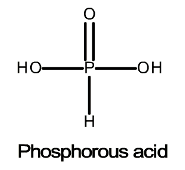# Problem: Phosphorous acid, H3PO4(aq), is a diprotic oxyacid that is an important compound in industry and agriculture.pKa1 = 1.30pKa2 = 6.70Calculate the pH for each of the following points in the titration of 50.0 mL of a 1.5 M H3PO3(aq) with 1.5 M KOH(aq).Part E. after addition of 100.0 mL of KOH

###### FREE Expert Solution

Calculate the volume needed to reach the equivalence point:

VB = 50.0 mL

To reach the 2nd equivalence point, we will need another 50.00 mL or a total of 100 mL of the base. Therefore, we reached the 2nd equivalence point.

After the first equivalence point, we will have H2PO3-. Write the chemical equation for the reaction between H2PO3- and NaOH.

Reaction:

H2PO3-(aq) + NaOH(aq) ⇌  HPO32-(aq) + H2O(l)
*Main group metals should have a +3 charge or higher to be considered acidic. Na+ has only a +1 charge and is a neutral ion so it will not contribute to the pH of the solution

Calculate the initial amounts of H2PO3- and NaOH in moles before the reaction happens.

82% (136 ratings)###### Problem Details

Phosphorous acid, H3PO4(aq), is a diprotic oxyacid that is an important compound in industry and agriculture.pKa1 = 1.30
pKa2 = 6.70

Calculate the pH for each of the following points in the titration of 50.0 mL of a 1.5 M H3PO3(aq) with 1.5 M KOH(aq).

Part E. after addition of 100.0 mL of KOH

Frequently Asked Questions

What scientific concept do you need to know in order to solve this problem?

Our tutors have indicated that to solve this problem you will need to apply the Titrations of Diprotic and Polyprotic Acids concept. You can view video lessons to learn Titrations of Diprotic and Polyprotic Acids. Or if you need more Titrations of Diprotic and Polyprotic Acids practice, you can also practice Titrations of Diprotic and Polyprotic Acids practice problems.